﻿ 直线电机外骨骼被动元件参数最优匹配方法
«上一篇文章快速检索 高级检索

 哈尔滨工程大学学报2019, Vol. 40Issue (8): 1488-1494, 1516  DOI: 10.11990/jheu.2018060450

### 引用本文DENG Jing, ZHA Fusheng, WANG Yuntao, et al. Method for optimizing the param-pattern of passive elements in the energy-efficient actuators of exoskeletons driven by linear motors[J]. Journal of Harbin Engineering University, 2019, 40(8), 1488-1494, 1516. DOI: 10.11990/jheu.201806045.### 文章历史

1. 哈尔滨工业大学 机器人技术与系统国家重点实验室, 黑龙江 哈尔滨 150001;
2. 深圳航天科技创新研究院, 广东 深圳 518057

Method for optimizing the param-pattern of passive elements in the energy-efficient actuators of exoskeletons driven by linear motors
DENG Jing 1, ZHA Fusheng 1, WANG Yuntao 2, ZHENG Chao 1, LI Mantian 1
1. State Key Laboratory of Robotics and System, Harbin Institute of Technology, Harbin 150001, China;
2. Shenzhen Academy of Aerospace Technology, Shenzhen 518057, China
Abstract: The energy consumption and duration of flight are key performance indexes used to determine the feasibility of using exoskeleton robots in practical applications. This work proposed a method for the optimization of the param-pattern of passive elements to reduce energy consumption by exoskeleton actuators. First, the object function and the constraint equation of the optimal param-pattern were proposed. Then, the optimal parameters of the passive element were identified by using an improved genetic algorithm based on the Kalman filtering algorithm. Experiments showed that this method can decrease energy consumption by exoskeleton actuators driven by linear motors.
Keywords: exoskeleton    passive element    energy-efficient    param-pattern    optimal problem

1 优化目标与约束条件 1.1 被动元件参数最优匹配优化目标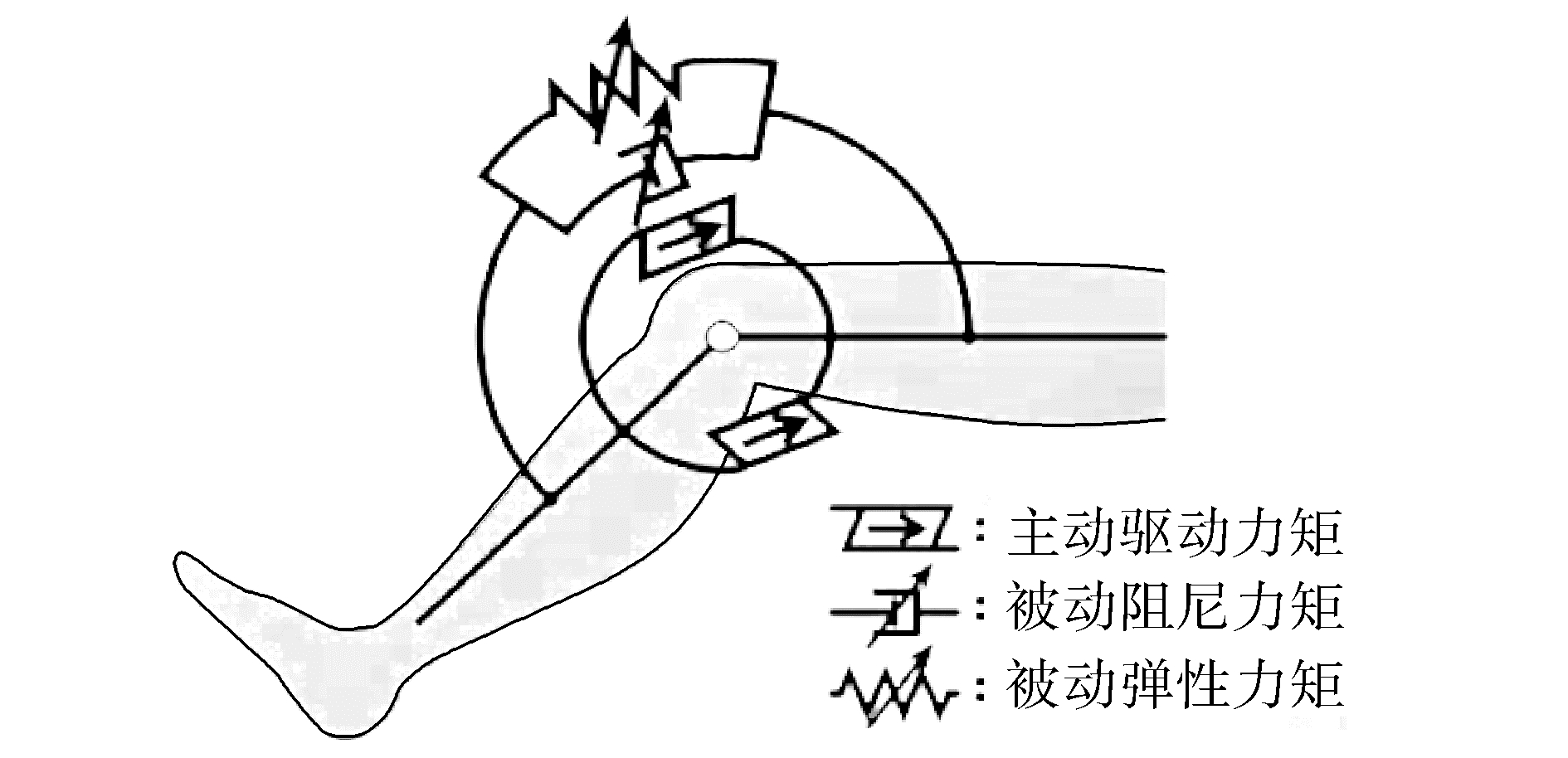Download: 图 1 外骨骼主-被动元件并联驱动示意 Fig. 1 Exoskeleton active-passive element parallel driver

 $P_{\mathrm{all}}=\int_{0}^{T} \sum\limits_{i=1}^{6}\left|\tau_{i}(t) \omega_{i}(t)\right| \mathrm{d} t$ (1)

 $T_{a i}=\tau_{i}+T_{k i}+T_{c i}$ (2)

 ${\rm{object}}\;\;{\rm{Function}} \to {\mathop{\rm Min}\nolimits} \left( {{P_{{\rm{ all }}}}} \right)$ (3)

1.2 被动元件参数最优匹配约束条件

 $P_{k i}=\int_{0}^{T}\left|T_{k i}(t) \omega_{i}(t)\right| \mathrm{d} t=0$ (4)

 $T_{k i}=\sum\limits_{k=0}^{n} a_{k} \theta_{i}^{k}$ (5)

 $P_{k i}=\int_{0}^{T}\left|\left(\sum\limits_{k=0}^{n} a_{k} \theta_{i}^{k}\right) \cdot \omega_{i}(t)\right| \mathrm{d} t=0$ (6)

 $\left\{ \begin{array}{l} T{c_i}\left( t \right) = - {b_i}\left( t \right) \cdot {\mathop{\rm sgn}} \left( {{\omega _i}} \right)\\ {\mathop{\rm sgn}} \left( {{\omega _i}} \right) = \left\{ {\begin{array}{*{20}{c}} {1, }&{{\omega _i} > 0}\\ {0, }&{{\omega _i} = 0}\\ { - 1, }&{{\omega _i} < 0} \end{array}} \right. \end{array} \right.$ (7)

 $\left\{ {\begin{array}{*{20}{l}} {{\tau _{i\min }} \le {\tau _i} \le {\tau _{i\max }}}\\ {{T_{ki\min }} \le {T_{ki}} \le {T_{ki\max }}}\\ {{T_{ci\min }} \le {T_{ci}} \le {T_{cimax}}} \end{array}} \right.$ (8)
2 被动元件参数最优匹配方法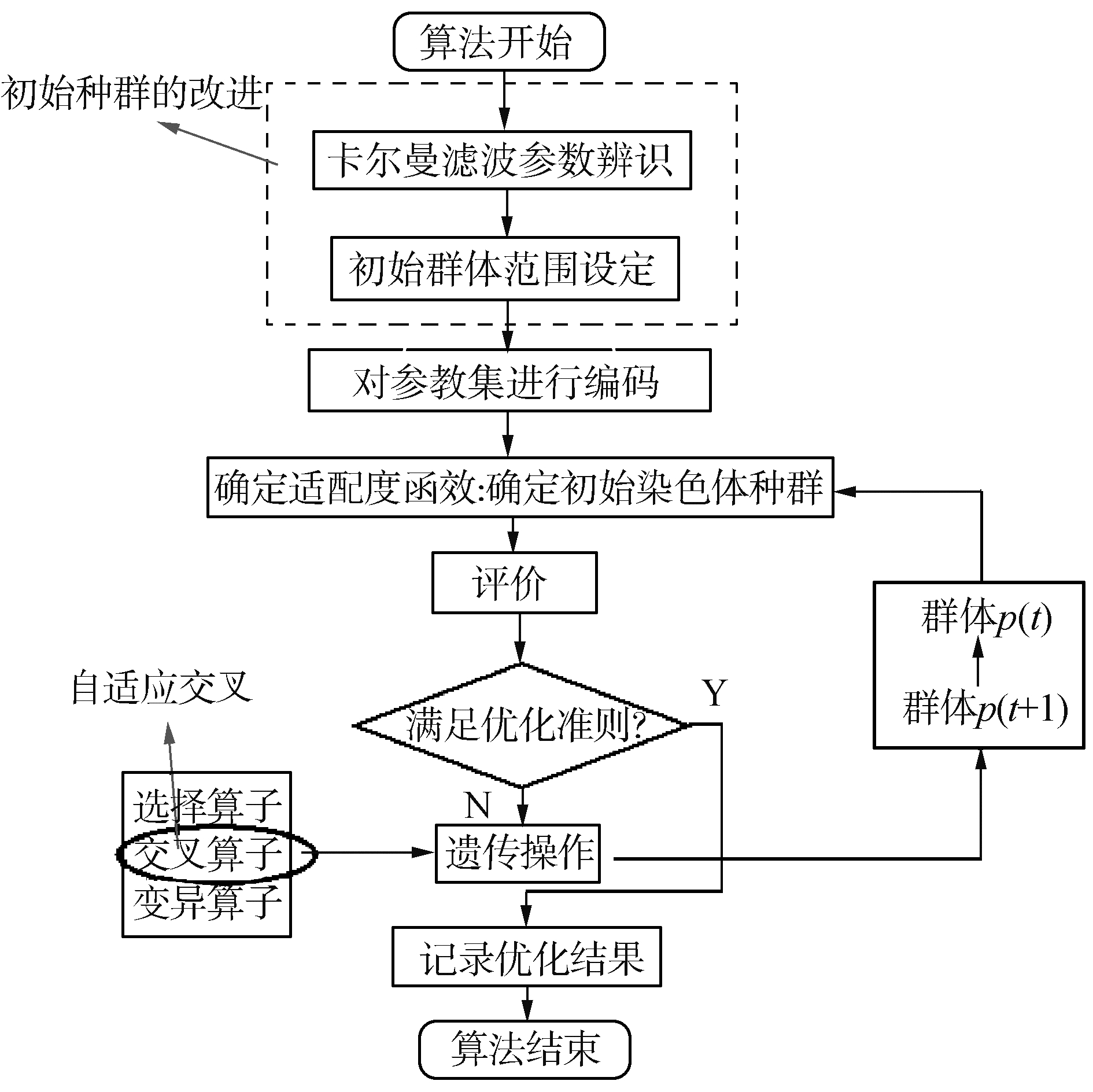Download: 图 2 改进遗传算法流程 Fig. 2 The flow chart of improved genetic algorithm

2.1 编码方式

 $\boldsymbol{p}=\left[\begin{array}{lllllllll}{p_{1}} & {\cdots} & {p_{n}} & {p_{n+1}} & {\cdots} & {p_{n+j}} & {\cdots} & {p_{n+m}}\end{array}\right]$ (9)

2.2 种群初始化

1) 将期望关节合力矩存入数据库中；

2) 通过卡尔曼滤波对数据库中的关节合力进行参数辨识，得到被动弹性力矩的泰勒展开多项式系数，即p1p2，…，pn的值；

3) 将p1p2pn的值域范围进行二次分配，pimax_newpimin_new为二次分配时值域的上下限：

 $\left\{ {\begin{array}{*{20}{c}} {{p_{i\max \_{\rm{new}}}} = {p_i} - {k_0}, }&{1 \le i \le n}\\ {{p_{i\min \_{\rm{new}}}} = {p_i} + {k_0}, }&{1 \le i \le n} \end{array}} \right.$ (10)

4) 选择pn+1pn+jpn+m的值域范围[0, fjmax]，在[0, fjmax]依次对第j项阻尼力矩随机二次分配。

2.3 适应度函数

 $\begin{array}{*{20}{c}} {{\mathop{\rm eval}\nolimits} (p) = \min \sum\limits_{j = 1}^m {\left( {|\tau (j) - \sum\limits_{i = 1}^n {{p_i}} \theta (j) - } \right.} }\\ {{p_{n + i}}{\mathop{\rm sgn}} (\omega (j)) \cdot \omega (j)| \cdot \Delta t)} \end{array}$ (11)

2.4 遗传操作

2.4.1 选择

 $\operatorname{prob}\left(p_{i}\right)=\operatorname{eval}\left(p_{i}\right) / \sum\limits_{j=1}^{n} \operatorname{eval}\left(p_{j}\right)$ (12)

2.4.2 交叉

 ${P_c} = - \left( {{P_{c{\rm{\_start}}}} - {P_{c{\rm{\_end}}}}} \right) \cdot {t^2}/{T^2} + {P_{c{\rm{\_start}}}}$ (13)

2.4.3 变异

 $\left\{ \begin{array}{l} p_i^{i + 1} = p_i^i + \left( {{p_{i\max }} - p_i^i} \right)c, \;\;\;\;\;w \ge 0.5\\ p_i^{i + 1} = p_i^i - \left( {p_i^i - {p_{i\min }}} \right)c, \;\;\;\;\;w < 0.5 \end{array} \right.$ (14)

2.5 终止条件

3 膝关节被动元件参数最优匹配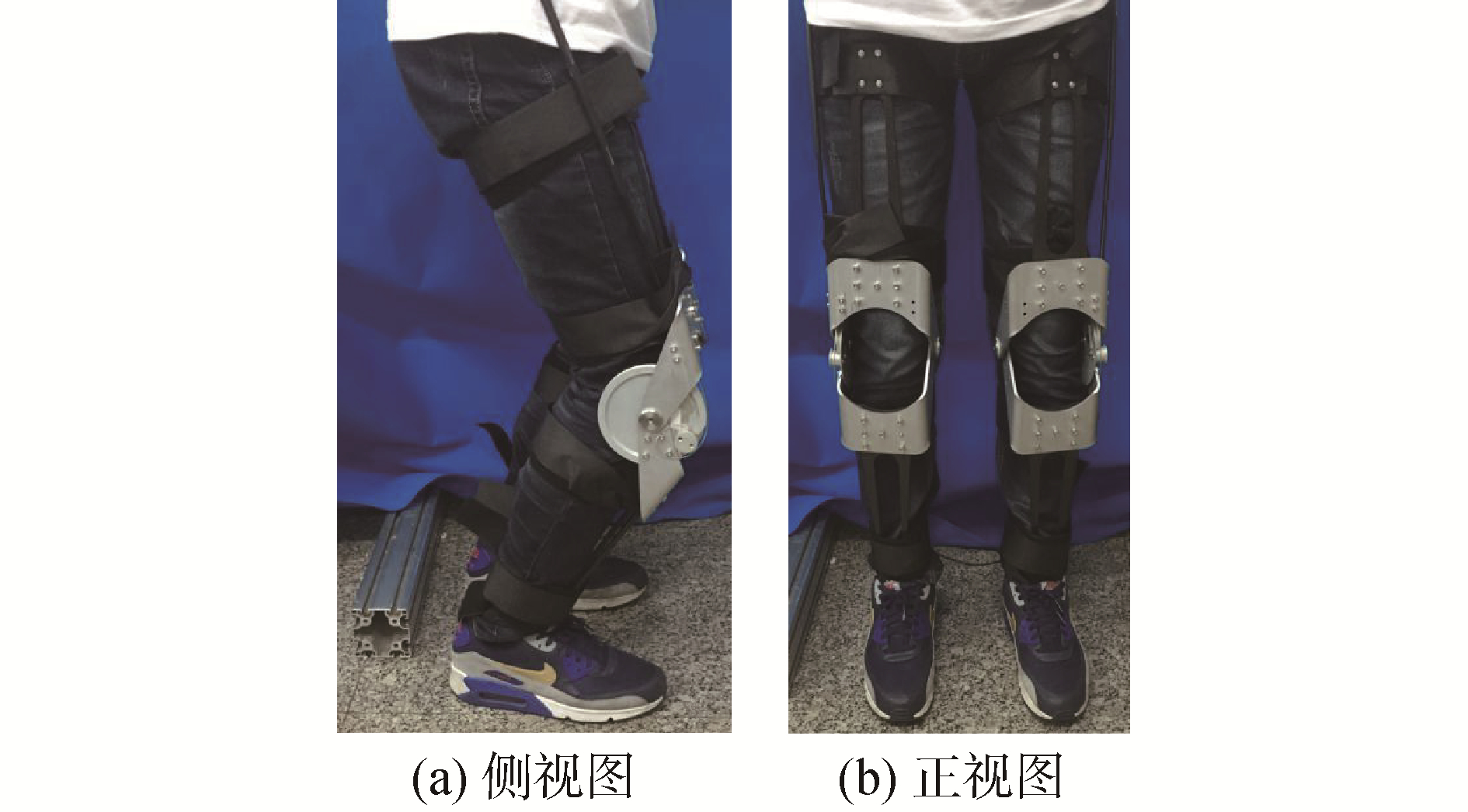Download: 图 3 直线电机驱动膝关节外骨骼 Fig. 3 Knee exoskeleton driven by linear motor表 1 改进遗传算法参数设定值 Table 1 Parameters of improved genetic algorithm表 2 多项式系数与最优能耗 Table 2 Polynomial coefficient and optimal energy consumption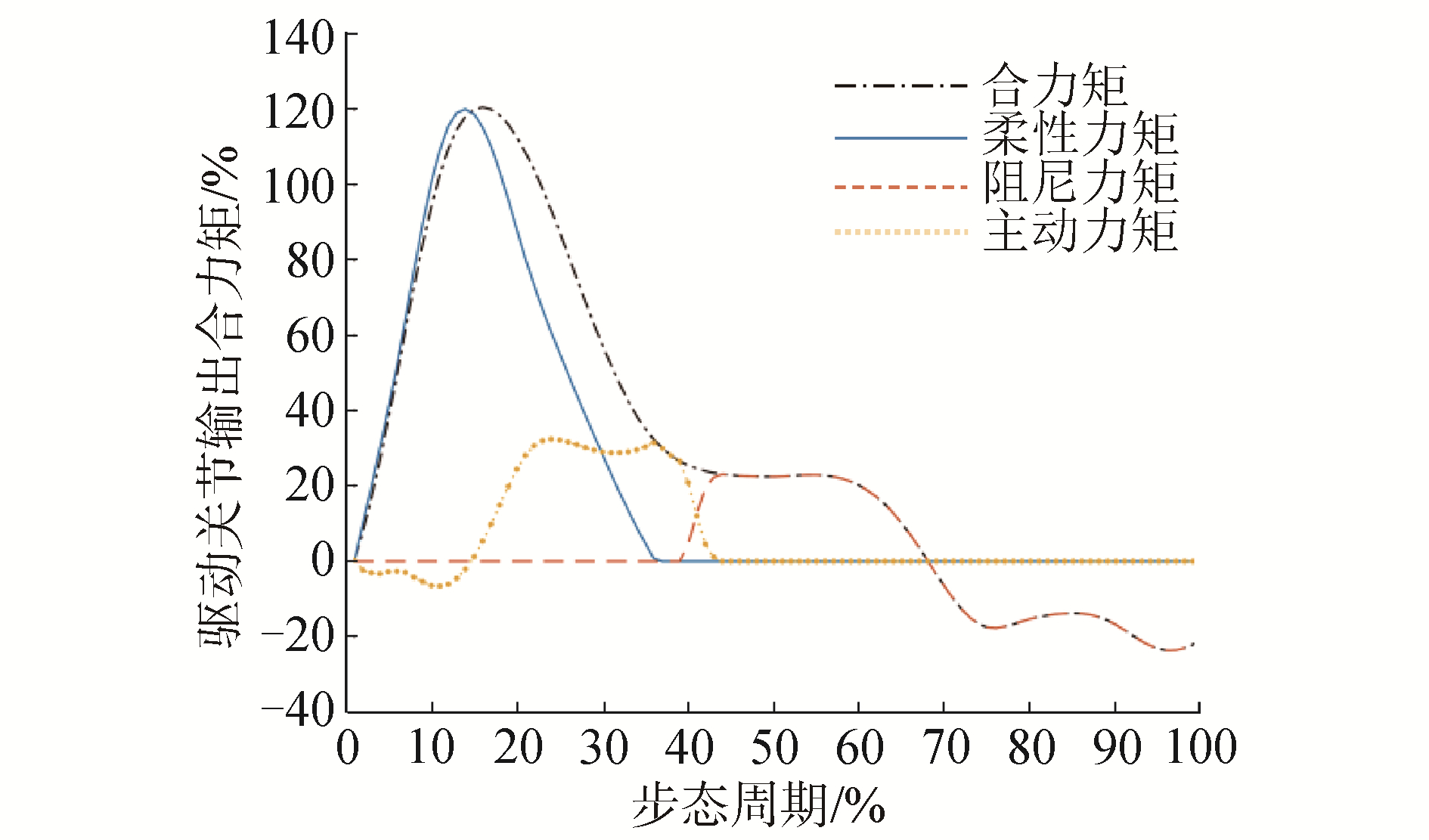Download: 图 4 膝关节主-被动力矩重构 Fig. 4 Reconstruction of the active & passive knee torque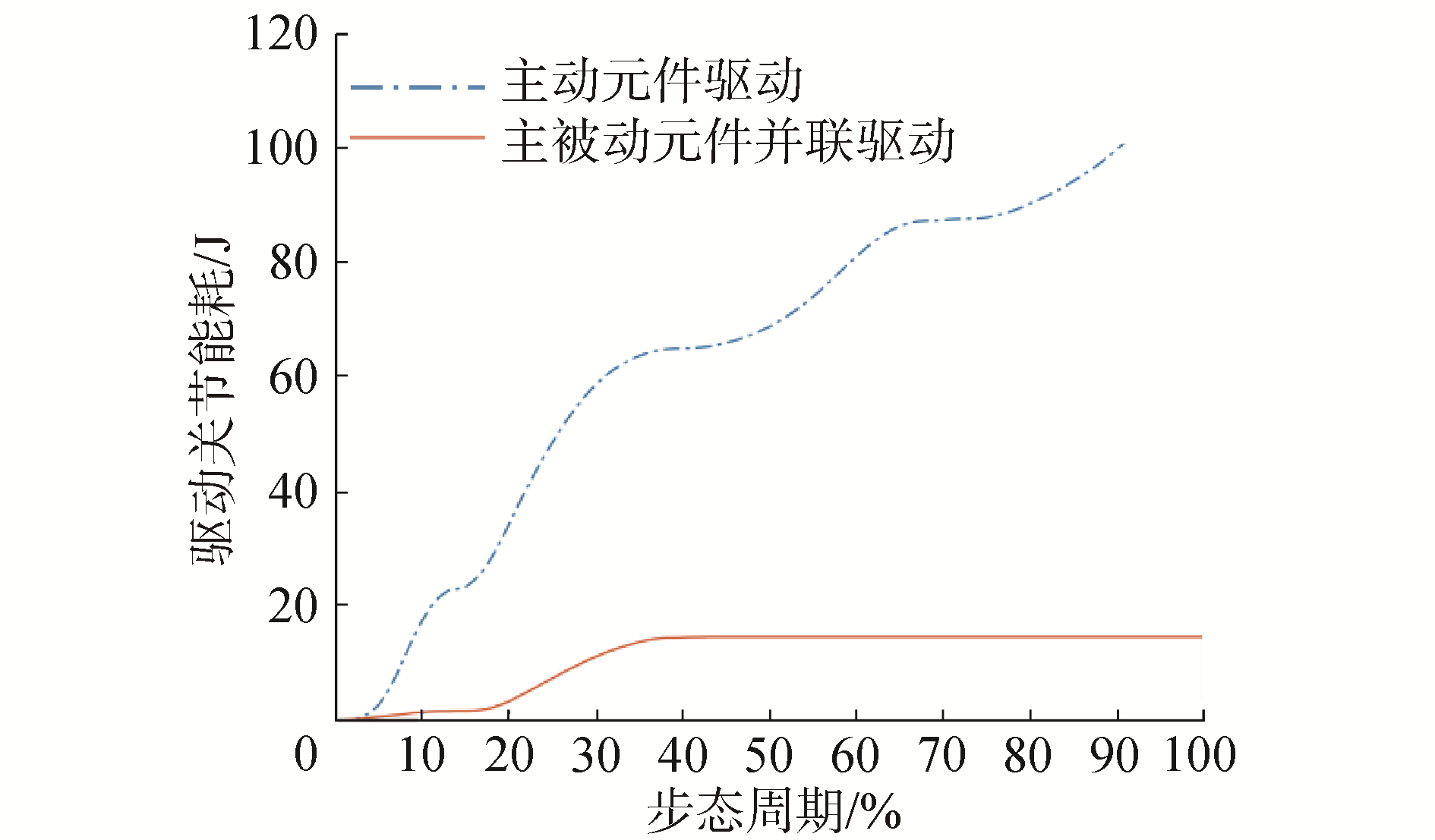Download: 图 5 膝关节驱动能耗对比 Fig. 5 Comparison of the energy consumption of knee driver
4 被动元件参数最优匹配实验Download: 图 6 主-被动并联驱动单元对顶实验平台 Fig. 6 Experimental platform of active-passive parallel driver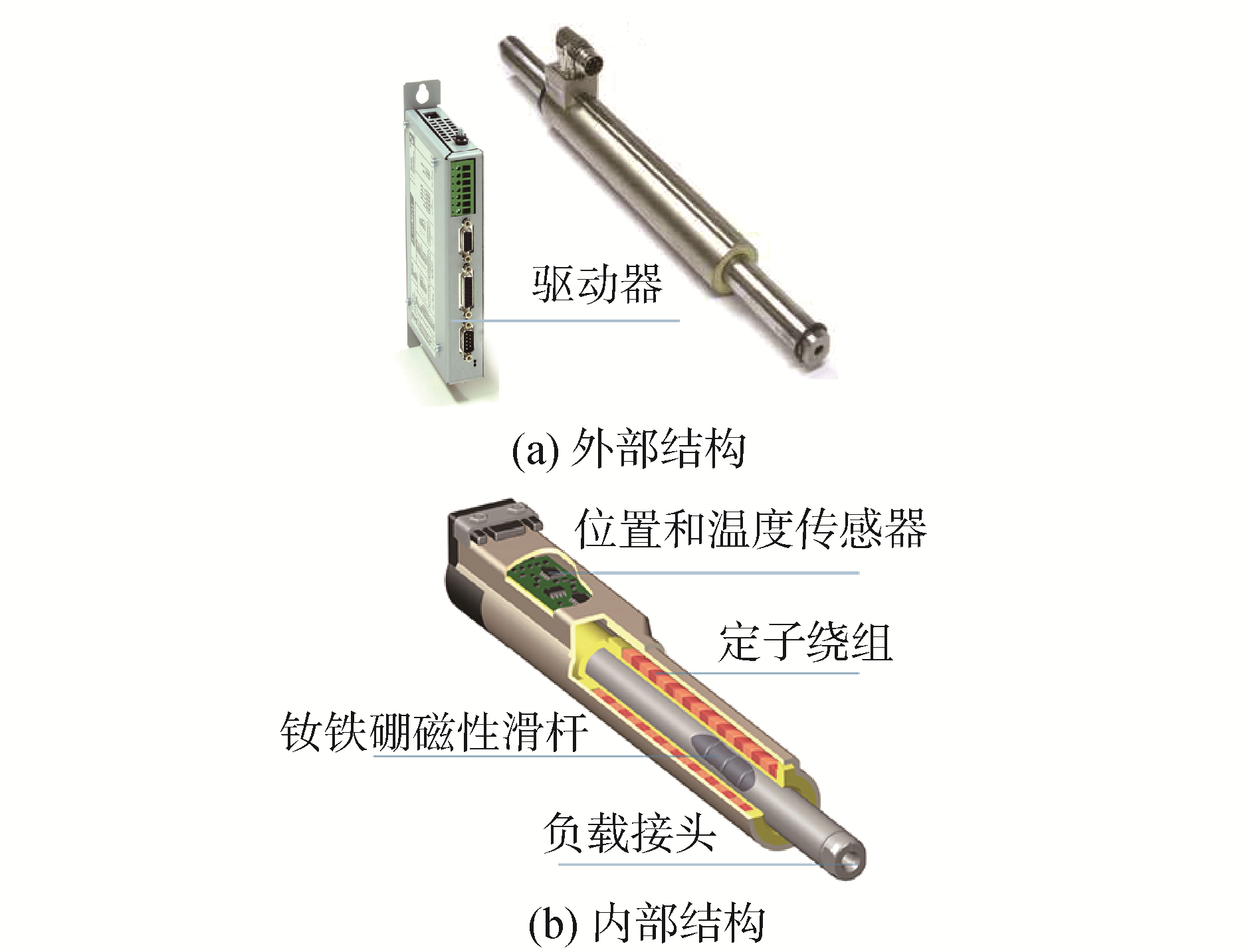Download: 图 7 直线电机结构 Fig. 7 The structure of linear motor

 $x=\theta \cdot e$ (15)

2组对照试验的关键是保证试验台右半部分的输出合力和位移与期望值相等，图 8为主-被动并联驱动单元对顶实验平台直线电机输出位移对期望位置的跟踪控制结果，图 9为直线电机输出力对期望力的跟踪控制结果。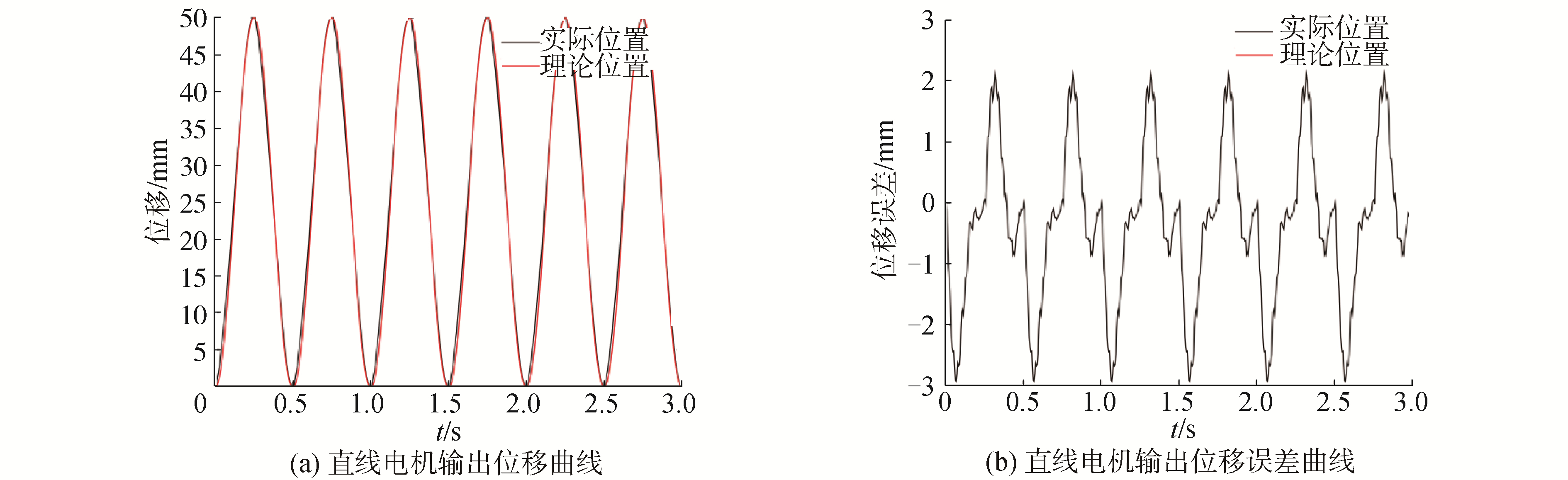Download: 图 8 直线电机位置跟踪控制结果 Fig. 8 The position tracking control property of linear motor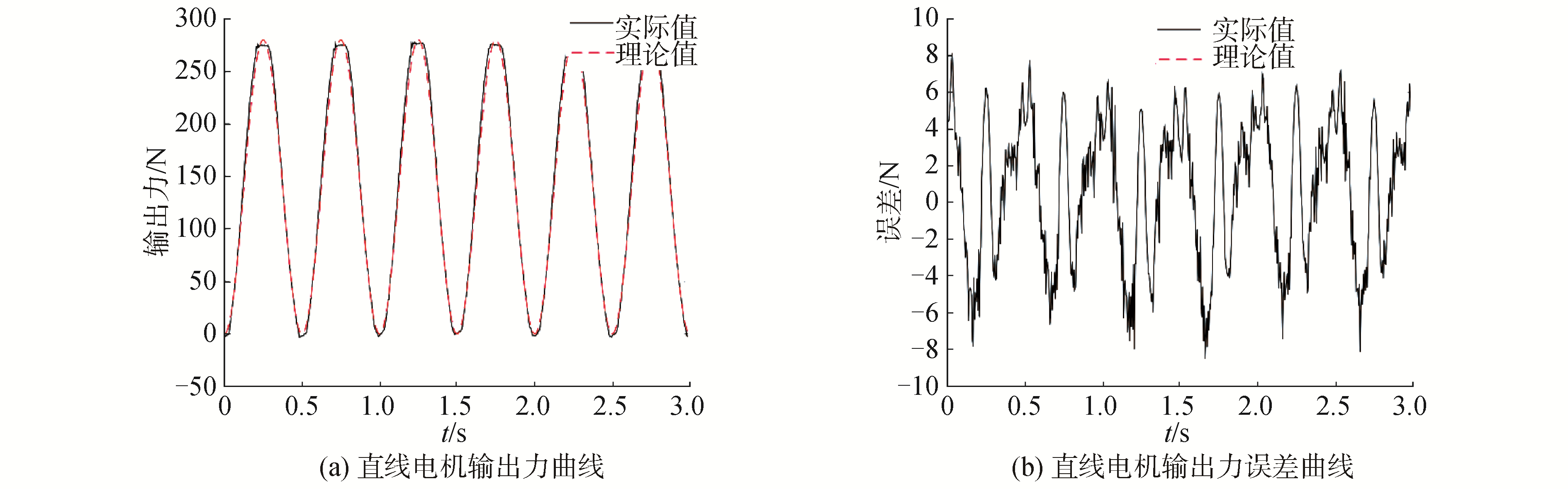Download: 图 9 直线电机力跟踪控制结果 Fig. 9 The force tracking control property of linear motor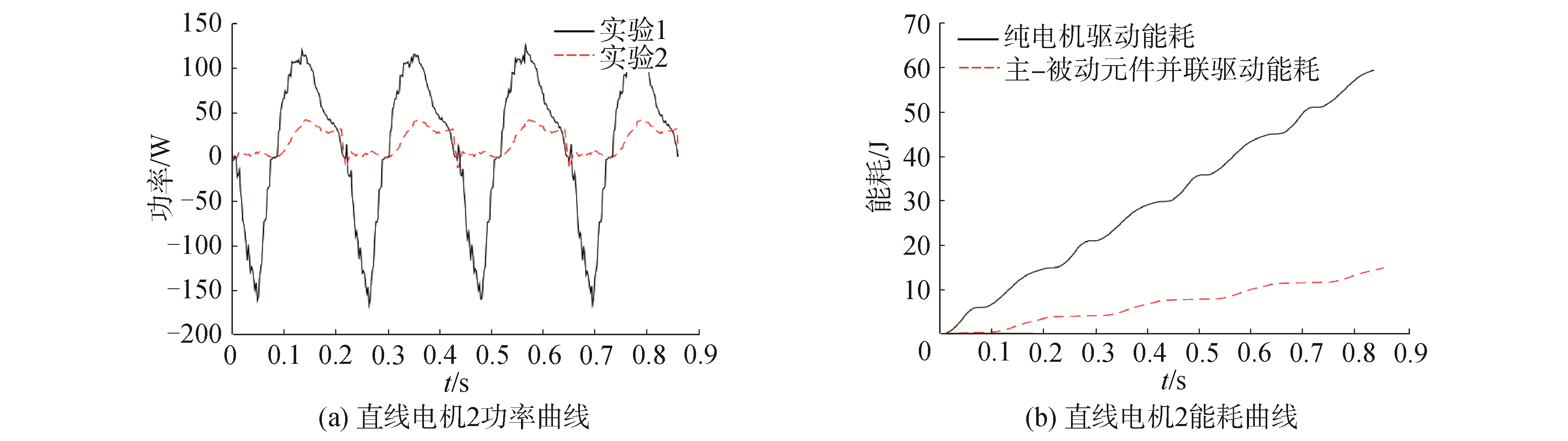Download: 图 10 实验一和实验二驱动电机功率与能耗对比 Fig. 10 Comparison of power and energy consumption of two experiments

5 结论

1) 被动元件参数的最优匹配问题本质上是一个优化问题。优化目标是使驱动关节的能耗最低，约束条件是保证驱动关节的输出合力矩为期望值。被动元件参数最优匹配的目标是在保证驱动关节期望输出合力矩的条件下，通过最优的匹配主动驱动力矩、被动弹性力矩和被动阻尼力矩使驱动关节能耗最低。

2) 基于卡尔曼滤波的改进遗传算法能够求解被动元件参数最优匹配问题。首先利用卡尔曼滤波生成较优的初始种群，使个体尽可能落在可行域内，从而减少无效个体产生的数量；其次利用自适应交叉概率，保证较优的个体能有较大的概率遗传到下一代。

3) 实验表明利用改进遗传算法求出的被动元件最优参数匹配，能够显著降低膝关节外骨骼驱动关节的能耗。

  KAZEROONI H, RACINE J L, HUANG Lihua, et al. On the control of the berkeley lower extremity exoskeleton (BLEEX)[C]//Proceedings of the 2005 IEEE International Conference on Robotics and Automation. Barcelona, Spain, 2005: 4356-4360. (0)  STEGER R, KIM S H, KAZEROONI H. Control scheme and networked control architecture for the Berkeley lower extremity exoskeleton (BLEEX)[C]//Proceedings of 2006 IEEE International Conference on Robotics and Automation, 2006. Orlando, FL, USA, 2006: 3469-3476. (0)  ZOSS A, KAZEROONI H. Architecture and hydraulics of a lower extremity exoskeleton[C]//Proceedings of ASME 2005 International Mechanical Engineering Congress and Exposition. Orlando, Florida, USA, 2005: 1447-1455. (0)  LOW K H, LIU Xiaopeng, YU Haoyong. Development of NTU wearable exoskeleton system for assistive technologies[C]//Proceedings of IEEE International Conference Mechatronics and Automation. Niagara Falls, Ont., Canada, 2005: 1099-1106. (0)  HIROSE S, KATO K. Study on quadruped walking robot in Tokyo institute of technology-past, present and future[C]//Proceedings of IEEE International Conference on Robotics and Automation. San Francisco, CA, USA, 2000: 414-419. (0)  BOBBERT M F, YEADON M R, NIGG B M. Mechanical analysis of the landing phase in heel-toe running[J]. Journal of biomechanics, 1992, 25(3): 223-234. (0)  COLLINS S H, WIGGIN M B, SAWICKI G S. Reducing the energy cost of human walking using an unpowered exoskeleton[J]. Nature, 2015, 522(7555): 212-215. DOI:10.1038/nature14288 (0)  WALSH C J, PASCH K, HERR H. An autonomous, underactuated exoskeleton for load-carrying augmentation[C]//Proceedings of 2006 IEEE/RSJ International Conference on Intelligent Robots and Systems. Beijing, China, 2007: 1410-1415. (0)  SANKAI Y. The leading edge of future technology "Cybernics": project HAL-toward robot suits and cyber suits?[C]//Proceedings of the Ninth IEEE International Symposium on Wearable Computers. Osaka, Japan, 2005: xvii. (0)  Spingwalker[EB/OL]. (2012). http://www.springwalker.com. (0)  EIBEN A E, SCHUT M C, DE WILDE A R. Boosting genetic algorithms with self-adaptive selection[C]//Proceedings of 2006 IEEE International Conference on Evolutionary Computation. Vancouver, BC, Canada, 2006: 477-482. (0)  王皓, 高立群, 欧阳海滨. 多种群随机差分粒子群优化算法及其应用[J]. 哈尔滨工程大学学报, 2017, 38(4): 652-660. WANG Hao, GAO Liqun, OUYANG Haibin. Multi-population random differential Particle swarm optimization and its application[J]. Journal of Harbin Engineering University, 2017, 38(4): 652-660. (0)  王国夫, 王鹢, 孙尧, 等. 混合GA与SA求解非线性约束优化[J]. 哈尔滨工程大学学报, 2002, 23(6): 73-76. WANG Guofu, WANG Yi, SUN Yao, et al. Hybrid GA and SA for solving nonlinear constrained optimization problems[J]. Journal of Harbin Engineering University, 2002, 23(6): 73-76. DOI:10.3969/j.issn.1006-7043.2002.06.019 (0)  YANG Yan, KAMEL M S. An aggregated clustering approach using multi-ant colonies algorithms[J]. Pattern recognition, 2006, 39(7): 1278-1289. DOI:10.1016/j.patcog.2006.02.012 (0)  COOK D F, RAGSDALE C T, MAJOR R L. Combining a neural network with a genetic algorithm for process parameter optimization[J]. Engineering applications of artificial intelligence, 2000, 13(4): 391-396. DOI:10.1016/S0952-1976(00)00021-X (0)